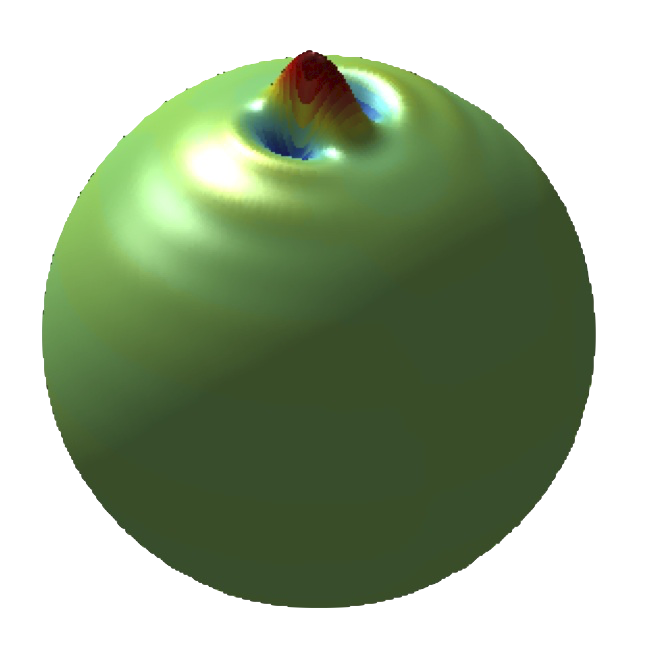# S2DW: Steerable scale discretised wavelets on the sphereS2DW provides functionality to perform the scale discretised wavelet transform on the sphere. Routines are provided to compute wavelet and scaling coefficients from the spherical harmonic coefficients of a signal on the sphere and to synthesise the spherical harmonic coefficients of the original signal from its wavelet and scaling coefficients. The reconstruction of the spherical harmonic coefficients of the original signal is exact to numerical precision.

Functionality to support functions defined on the sphere is provided by the S2 code.

S2DW has been effectively been superseeded by the S2LET code, which supports exact sampling theorems, a variety of modern programming languages and spin wavelets.